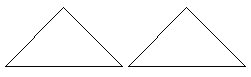Email us to get an instant 20% discount on highly effective K-12 Math & English kwizNET Programs!

#### Online Quiz (WorksheetABCD)

Questions Per Quiz = 2 4 6 8 10

### Grade 3 - Mathematics8.9 Congruent and Symmetric Figures

 When two figures are exactly the same, they are said to be congruent. When a figure can be folded into equal pieces, then it is said to be symmetric. The line along which the figure is folded is called the line of symmetry. Example: How many lines of symmetry does this figure have?Answer: 4 Directions: Answer the following. Also draw at least five symmetric figures and show their line of symmetry.
 Q 1: Two figures that are exactly the same shape and size are said to bedifferentsimilarcongruent Q 2: Are the two triangles in the figure congruent?TrueFalse Q 3: In a symmetrical figure the folded line is calledthe line of symmetryradiusdiameter Q 4: These two figers are congruent.FalseTrue Q 5: How many lines of symmetry does a square have?3124 Q 6: When a figure can be folded in half and both halves match the figure is said to beexact matchsymmetricaldifferent Question 7: This question is available to subscribers only! Question 8: This question is available to subscribers only!